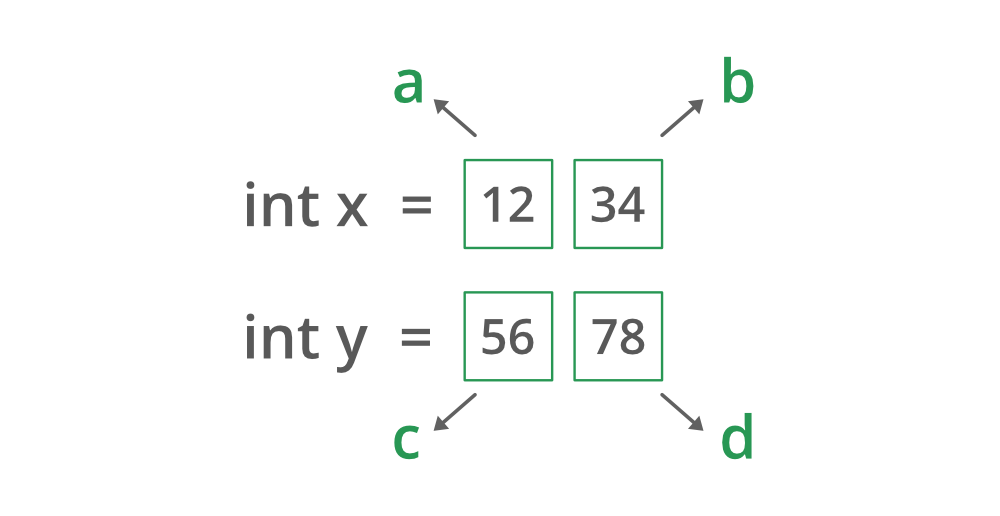Related Articles

# Java Program to Implement the Karatsuba Multiplication Algorithm

• Last Updated : 21 Aug, 2021

It is a replacement for the grade school algorithm for multiplying numbers. of bigger digits. The Karatsuba Algorithm for fast multiplication algorithm using Divide and Conquer developed by Anatolii Alexeevitch Karatsuba in 1960. The first thing that hits the head what is it and why it is designed. Though there are 3 ways to multiply numbers :

1. Third-grade school algorithm Method (Standard-way)
2. Recursive algorithm Method
3. Karatsuba Multiplication Method

Why the Karatsuba algorithm?

The goal of the algorithm is designed because the design space is surprisingly rich. Its time complexity is follows and do not it as time complexity for this is very important and is sometimes do asked in interview questions.

O(n^log2(3)) time (~ O(n^1.585))

Where n is the number of digits of the numbers multiplying. It is discussed by multiplying two big integer numbers to show internal working step by step. The goal is to reduce the space complexity for which the integer numbers terms will be broken down in such a way to x and y are broken into a set of digits as the logic behind it is divide and conquer. If the numbers are smaller there is no need to multiply, standard mutilation of two integers is preferred.

The algorithm is standardized for 4 digits for sake of understanding. One can multiply as many digits taken into sets.

Algorithm Steps:

1. Compute starting set  (a*c)
2. Compute set after starting set may it be ending set (b*d)
3. Compute starting set with ending sets
4. Subtract values of step 3 from step2 from step1
5. Pad up (Add) 4 zeros to the number obtained from Step1, step2 value unchanged, and pad up two zeros to value obtained from step4.

Now let us do propose the above steps and showing it with an illustration prior getting to the implementation part

Illustration:

`Input: x = 1234, y = 5678````Processing: As per above inputs

x = 1234
y = 5678

a = 12, b = 34
c = 56, d = 78

Step 1: a * c = 172
Step 2: b * d = 2652
Step 3: (a+b)(c+d) = 134 * 36 = 6164
Step 4: 6164 - 2652 - 172 = 2840
Step 5: 1720000 + 2652 + 284000 = 7006652```
`Output: 7006652`

The value obtained from step 5 is in fact the product obtained if standard school multiplication is carried on between these two numbers ‘x’ and ‘y’.

`1720000 + 2652 + 284000 = 7006652`

Implementation: Note not to grasp the formulas laid down for this algorithm rather understanding it in this way makes its way to better.

## Java

 `/// Java Program to Implement Karatsuba Algorithm` `// Importing Random class from java.util packahge``import` `java.util.Random;` `// MAin class``class` `GFG {` `    ``// Main driver method``    ``public` `static` `long` `mult(``long` `x, ``long` `y) {` `        ``// Checking only if input is within range ``        ``if` `(x < ``10` `&& y < ``10``) {``          ` `            ``// Multiplying the inputs entered``            ``return` `x * y;``        ``}``     ` `        ``// Declaring variables in order to ``        ``// Find length of both integer``        ``// numbers x and y``        ``int` `noOneLength = numLength(x);``        ``int` `noTwoLength = numLength(y);` `        ``// Finding maximum length from both numbers``        ``// using math library max function``        ``int` `maxNumLength``            ``= Math.max(noOneLength, noTwoLength);` `        ``// Rounding up the divided Max length``        ``Integer halfMaxNumLength``            ``= (maxNumLength / ``2``) + (maxNumLength % ``2``);` `        ``// Multiplier``        ``long` `maxNumLengthTen``            ``= (``long``)Math.pow(``10``, halfMaxNumLength);` `        ``// Compute the expressions``        ``long` `a = x / maxNumLengthTen;``        ``long` `b = x % maxNumLengthTen;``        ``long` `c = y / maxNumLengthTen;``        ``long` `d = y % maxNumLengthTen;`  `        ``// Compute all mutilpying variables``        ``// needed to get the multiplication   ``        ``long` `z0 = mult(a, c);``        ``long` `z1 = mult(a + b, c + d);``        ``long` `z2 = mult(b, d);` `        ``long` `ans = (z0 * (``long``)Math.pow(``10``, halfMaxNumLength * ``2``) +``                   ``((z1 - z0 - z2) * (``long``)Math.pow(``10``, halfMaxNumLength) + z2));` `        ``return` `ans;` `    ``}``  ` `      ``// Method 1``    ``// To calculate length of the number``    ``public` `static` `int` `numLength(``long` `n)``    ``{``        ``int` `noLen = ``0``;``        ``while` `(n > ``0``) {``            ``noLen++;``            ``n /= ``10``;``        ``}` `        ``// Returning length of number n``        ``return` `noLen;``    ``}` `     ``// Method 2``    ``// Main driver function``    ``public` `static` `void` `main(String[] args)``    ``{``        ``// Showcasing karatsuba multiplication``        ` `      ``// Case 1: Big integer lengths``        ``long` `expectedProduct = ``1234` `* ``5678``;``        ``long` `actualProduct = mult(``1234``, ``5678``);` `        ``// Printing the expected and corresponding actual product``        ``System.out.println(``"Expected 1 : "` `+ expectedProduct);``        ``System.out.println(``"Actual 1 : "` `+ actualProduct + ``"\n\n"``);``      ` `        ``assert``(expectedProduct == actualProduct);`  `        ``expectedProduct = ``102` `* ``313``;``        ``actualProduct = mult(``102``, ``313``);` `        ``System.out.println(``"Expected 2 : "` `+ expectedProduct);``        ``System.out.println(``"Actual 2 : "` `+ actualProduct + ``"\n\n"``);``        ` `      ``assert``(expectedProduct == actualProduct);` `        ``expectedProduct = ``1345` `* ``63456``;``        ``actualProduct = mult(``1345``, ``63456``);` `        ``System.out.println(``"Expected 3 : "` `+ expectedProduct);``        ``System.out.println(``"Actual 3 : "` `+ actualProduct + ``"\n\n"``);``        ` `      ``assert``(expectedProduct == actualProduct);       ``    ` `        ``Integer x = ``null``;``        ``Integer y = ``null``;``        ``Integer MAX_VALUE = ``10000``;` `        ``// Boe creating an object of random class``        ``// inside main() method``        ``Random r = ``new` `Random();` `        ``for` `(``int` `i = ``0``; i < MAX_VALUE; i++) {``            ``x = (``int``) r.nextInt(MAX_VALUE);``            ``y = (``int``) r.nextInt(MAX_VALUE);` `            ``expectedProduct = x * y;` `            ``if` `(i == ``9999``) {``              ` `              ``// Prove assertions catch the bad stuff.``                ``expectedProduct = ``1``;   ``            ``}``            ``actualProduct = mult(x, y);` `             ``// Again printing the expected and``            ``// corresponding actual product``            ``System.out.println(``"Expected: "` `+ expectedProduct);``            ``System.out.println(``"Actual: "` `+ actualProduct + ``"\n\n"``);` `            ``assert``(expectedProduct == actualProduct);       ``        ``}``    ``}``}`
Output
```Product 1 : 85348320
Product 2 : 21726```

Attention reader! Don’t stop learning now. Get hold of all the important Java Foundation and Collections concepts with the Fundamentals of Java and Java Collections Course at a student-friendly price and become industry ready. To complete your preparation from learning a language to DS Algo and many more,  please refer Complete Interview Preparation Course.

My Personal Notes arrow_drop_up